# How to Find the Perimeter of a Rectangle

Perimeter formulas, practice questions, and more.

Solving rectangular perimeter problems is a skill that has many interesting real-world applications. For example, we can use perimeter calculations for situations such as fencing requirements around a play field, measurements of a picture frame, distances around a walking path, or dimensions of a large window. Calculating the perimeter of rectangles is a helpful skill to master because it is used frequently in our daily lives.

Let’s remember that perimeter refers to the distance around the outside of a two-dimensional shape. It can be helpful to visualize the perimeter as the fencing that surrounds a park or a backyard. When we calculate the perimeter, we are essentially calculating the total distance around that two-dimensional shape.

Calculating perimeter problems can be done in many ways, but the most efficient strategy is to simply use the perimeter formula.

## What is the perimeter of a rectangle?

The perimeter formula for a rectangle states that P = (L + W) × 2, where P represents perimeter, L represents length, and W represents width. When you are given the dimensions of a rectangular shape, you can simply plug in the values of L and W into the formula in order to solve for the perimeter. For example, if the rectangle below represents a garden that needs a brick border, we can use the perimeter formula to determine how many feet of brick border we need in all.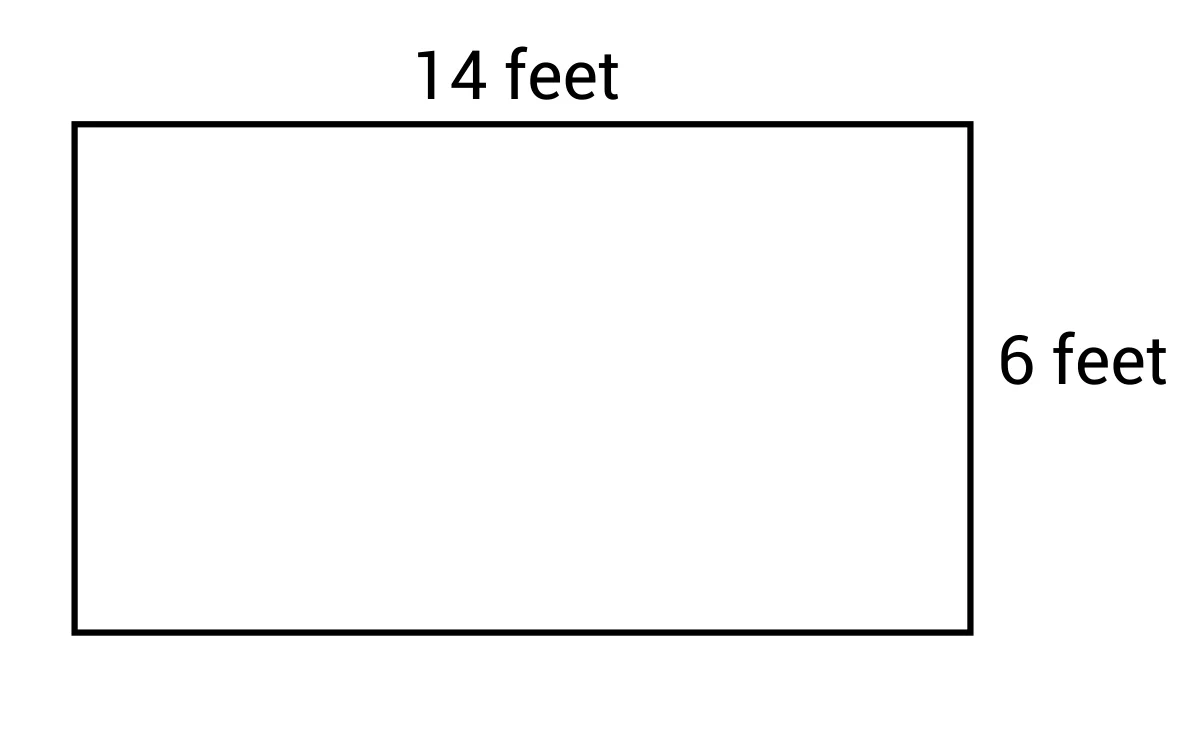### Perimeter of Rectangle Formula

The formula states that P = (L + W) × 2, so let’s plug in 14 feet for L and 6 feet for W. Now we have P = (14 + 6) × 2, which simplifies to 40, or 40 feet.

Using the perimeter formula saves us a bit of time by avoiding the alternative strategy which would be to add up each side length separately. 14 + 6 + 14 + 6 would produce the correct answer, but this strategy will generally take longer, so using the formula is recommended.

However, not all perimeter problems will provide you with the length and width in such a straightforward way. In fact, some perimeter problems will provide you with one dimension as well the rectangle’s area. In order to solve this kind of perimeter problem we need to review our understanding of area. Remember, to calculate the area of a rectangle, we simply multiply the length by the width. A rectangle that measures 3.5 cm by 4 cm would have an area of 14 cm2 because 3.5 × 4 = 14. Let’s use this knowledge of area and apply it to a perimeter problem.

For example, suppose you needed to frame a large rectangular window that has an area of 35 square feet and a length of 7 feet. Let’s use what we know about calculating area in order to find the perimeter.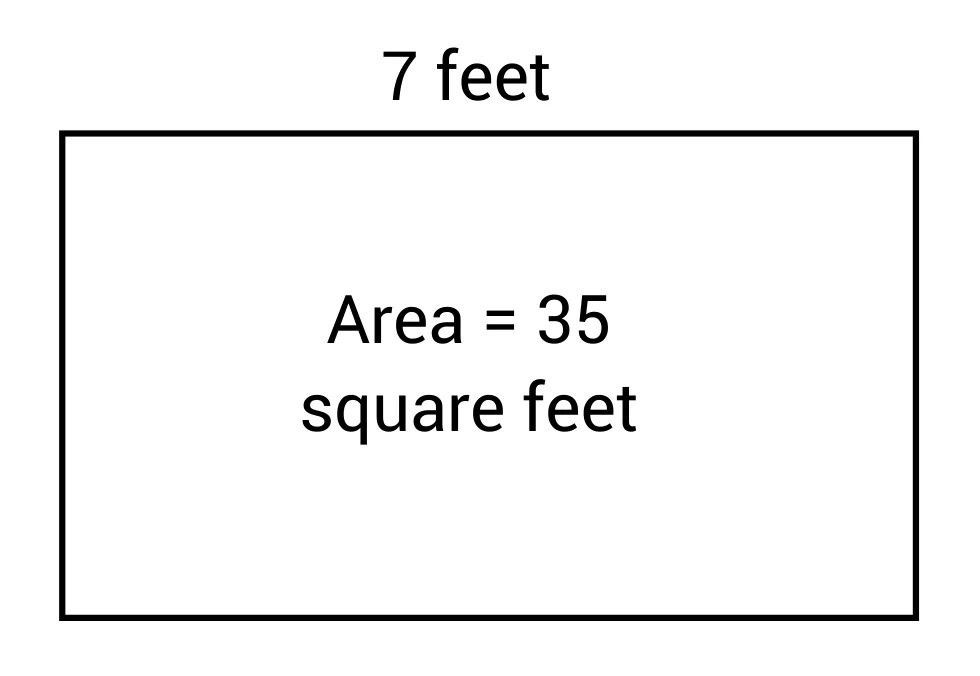We know that surface area is found by multiplying the length by the width. This means that seven multiplied by something equals 35, 7 × ? = 35. We can solve for the missing side length by dividing 35 by 7, which equals 5. We can now apply the perimeter formula because we know that the length is 7 feet and the width is 5 feet.

P = (L + W) × 2 becomes P = (7 + 5) × 2 which simplifies to 24, or 24 feet.

Now that we have reviewed the perimeter formula and its various applications, consider the following question. Does a rectangle with an area of 20 square feet have more than one possible option for its perimeter?

If you said yes, then you are correct. Rectangles with a restricted area such as 20 square feet can have a variety of perimeters. For example, a 20-square-foot rectangle could have the dimensions 1 ft × 20 ft, 2 ft × 10 ft, or 4 ft × 5 ft. All of these options will produce a different perimeter even though they all have the same area. Let’s prove this concept by calculating the perimeter of the three possible rectangles just mentioned: 1 ft × 20 ft, 2 ft × 10 ft, and 4 ft × 5 ft.

1 ft × 20 ft: P = (L + W) × 2 = (1 + 20) × 2 = 42 feet
2 ft × 10 ft = L + W × 2 = (2 + 10) × 2 = 24 feet
4 ft × 5 ft = L + W × 2 = 4 + 5 × 2 = 18 feet

It is also important to note that the formula P = (L + W) × 2 only applies to rectangles. This formula will only work for quadrilaterals that have two sets of congruent sides.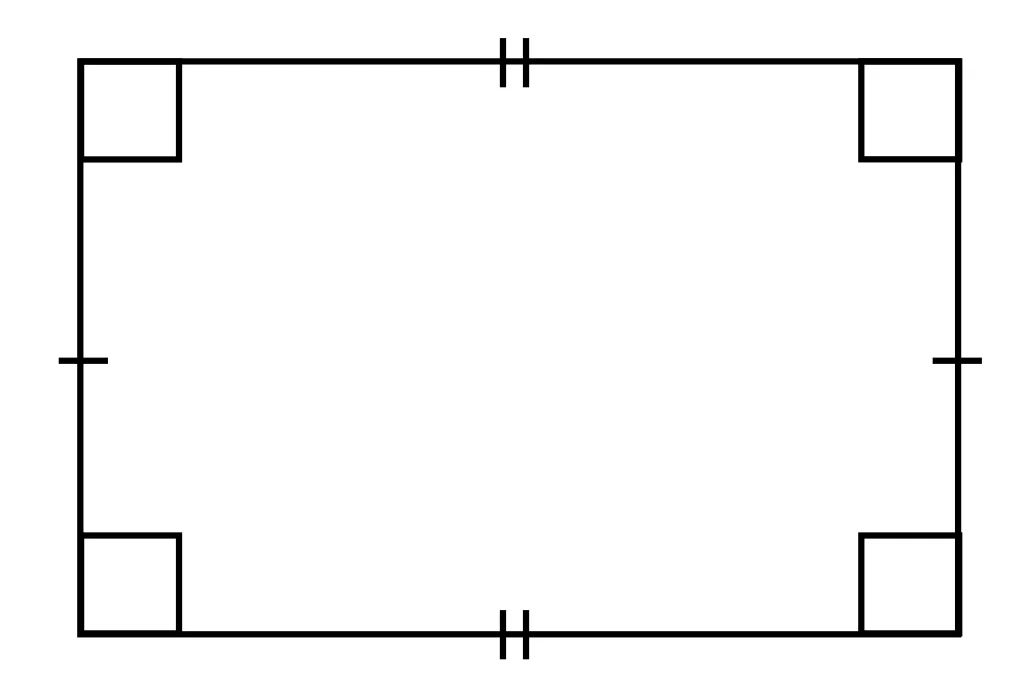## Perimeter of a Rectangle Sample Questions

Here are a few sample questions going over area of a rectangle.

Question #1:

Which formula is used to calculate the perimeter of a rectangle?

$$Perimeter=4+(length+width)$$
$$Perimeter=\frac{(\text{length }×\text{ width})}{2}$$
$$Perimeter=(length+width)×4$$
$$Perimeter=(length+width)×2$$

A rectangle has two equal lengths and two equal widths. In order to find the perimeter, or distance around the rectangle, we need to add up all four side lengths. This can be done efficiently by simply adding the length and the width, and then multiplying this sum by two since there are two of each side length. $$Perimeter=(length+width)×2$$ is the formula for perimeter.

Question #2:

Calculate the perimeter of the given rectangle.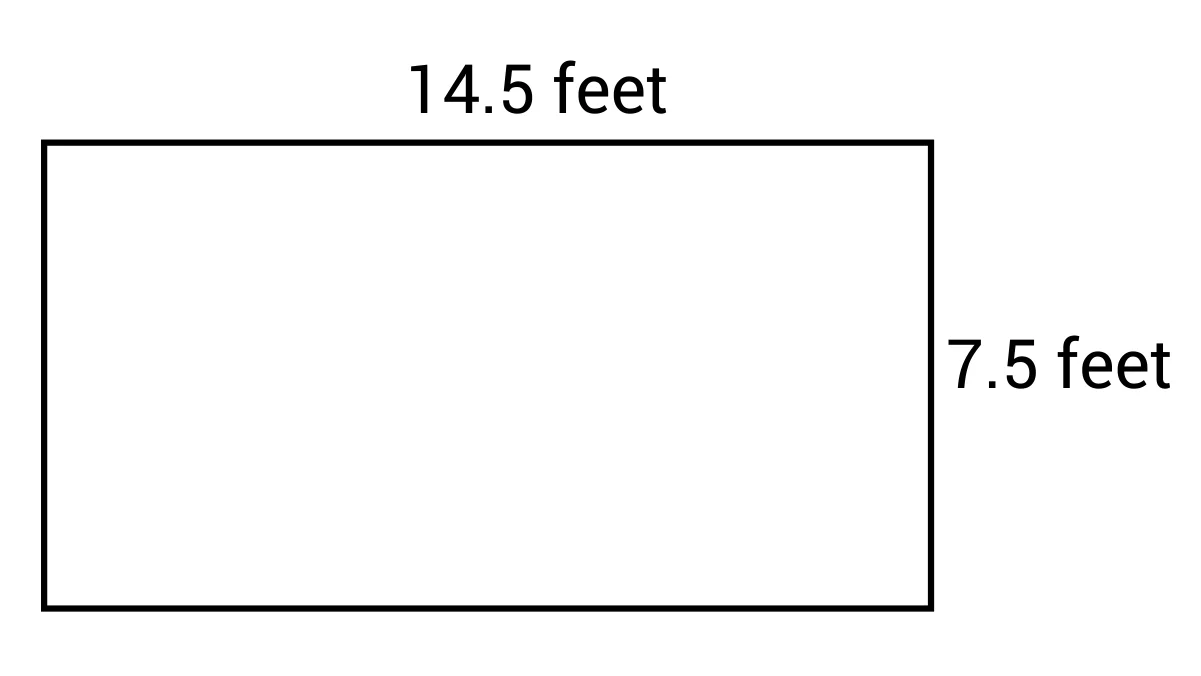22 feet

44 feet

86.5 feet

108.75 feet

We can calculate the perimeter of the rectangle by using the formula $$Perimeter=(length+width)×2$$. We can see that the length is 14.5 feet and the width is 7.5 feet so our formula becomes $$Perimeter=(14.5+7.5)×2$$ which simplifies to 44. The perimeter of the rectangle is 44 feet.

Question #3:

Determine the perimeter of the rectangle if its area is 64 m2 and its length is 16 m.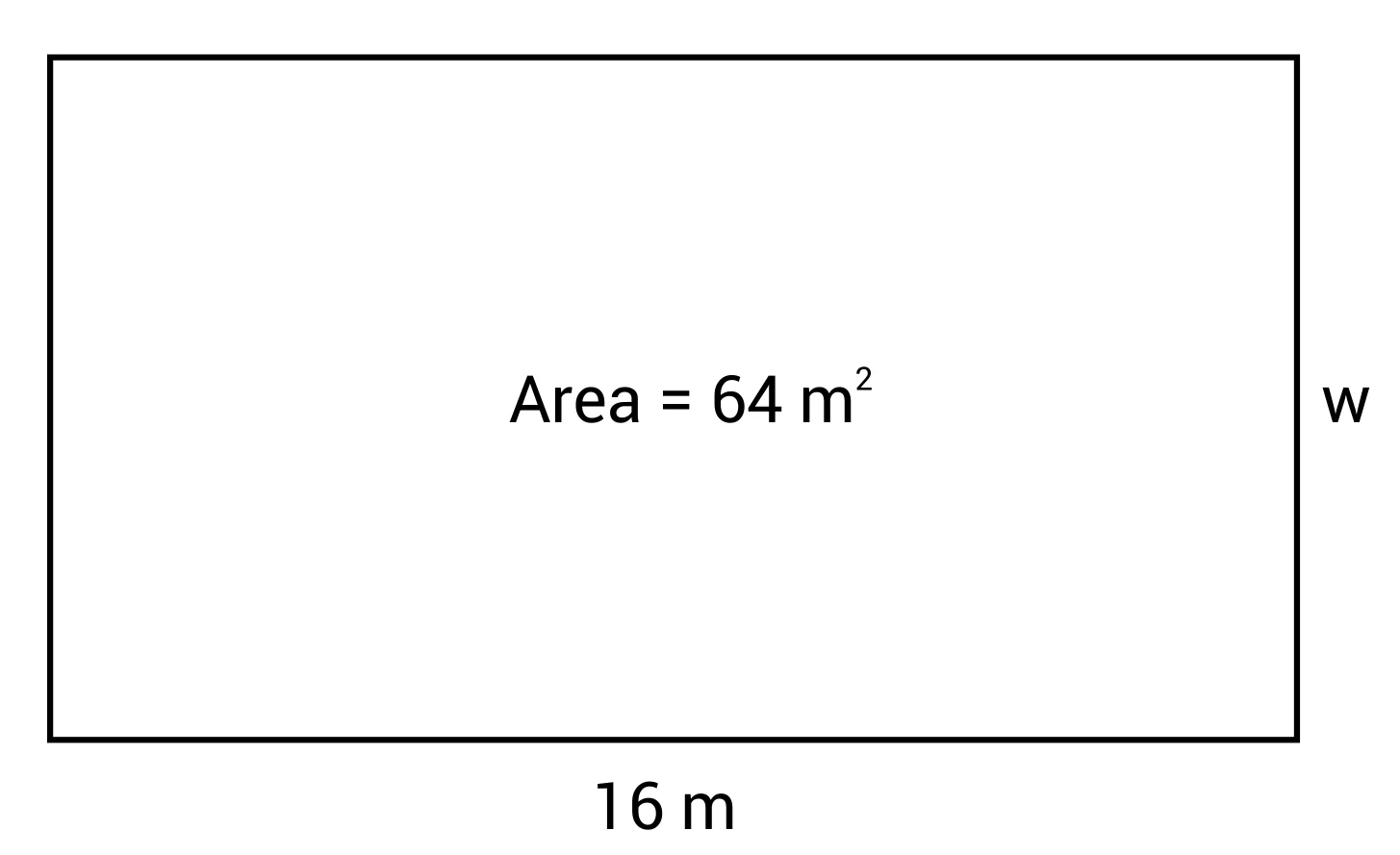40 meters

45 meters

30 meters

38 meters

In order to determine the perimeter of the rectangle, we first need to determine the width (w). The area of a rectangle is calculated by multiplying $$length×width$$, so we can use the following equation to solve for our missing value (w).

Area = l × w
64 m2=16 × w

Divide both sides by 16 to solve for w.

w = 4, or 4 m

Now that we know the length and the width, we can use the formula $$Perimeter=(length+width)×2$$ in order to solve for the perimeter. When we plug in 16 m for our length and 4 m for our width, we have the following: $$Perimeter=(16 m+4 m)×2$$, which simplifies to 40 m. The perimeter of the rectangle is 40 meters.

Question #4:

The side lengths of a rectangular sandbox in Sunnyland Park are 15 feet and 8.5 feet. Determine the perimeter of the sandbox.

47 feet

42 feet

46.5 feet

49.5 feet

We can calculate the perimeter of the sandbox by using the formula $$Perimeter=(length+width)×2$$. We know that the length is 15 feet and the width is 8.5 feet so we can plug these values into the formula in order to solve for Perimeter. Our formula now becomes $$Perimeter=(15+8.5)×2$$ which simplifies to 47, or 47 feet.

Question #5:

Gloria is designing a garden for her backyard. She knows that she wants to have 24 square feet in the garden, but she is flexible as far as what the dimensions are. She wants to put a fence around the garden, but fencing (per foot) can be quite expensive so she wants to compare options. Option A is to build a garden with dimensions of 8 feet by 3 feet. Option B is to build a garden with dimensions of 6 feet by 4 feet. Which option will require less fencing and therefore a lower cost?

Option A is less expensive

Option B is less expensive